# Class and object in C++

## 1- Introduction

This document is based on:

## 2- Note for Eclipse

If you work with:  Windows 64bit + Eclipse 64bit + Java64bit, you need to open Eclipse with Administrator right, there is trouble that  Eclipse does not print the message on the   Console screen in case of running in normal mode.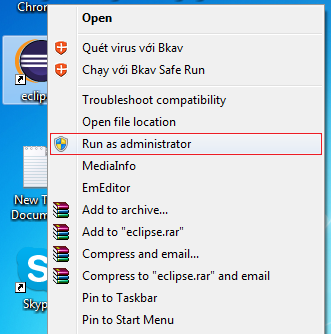## 3- Create C++ project on Eclipse

In Eclipse select:
• File/New/Other..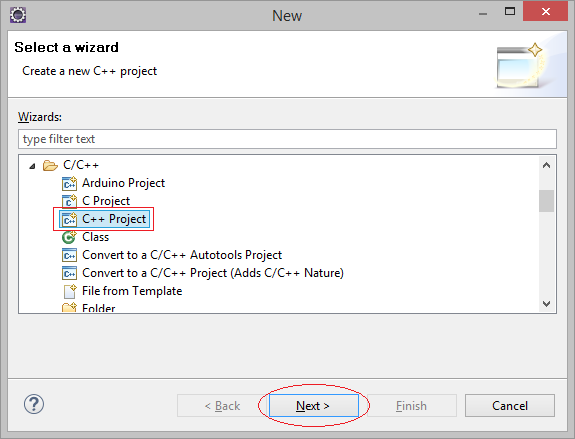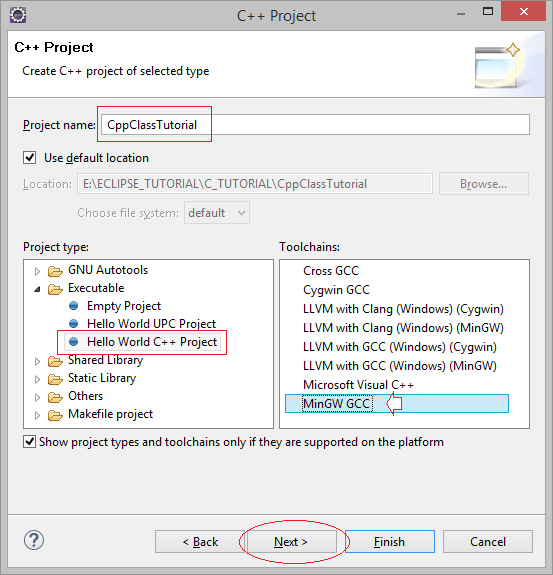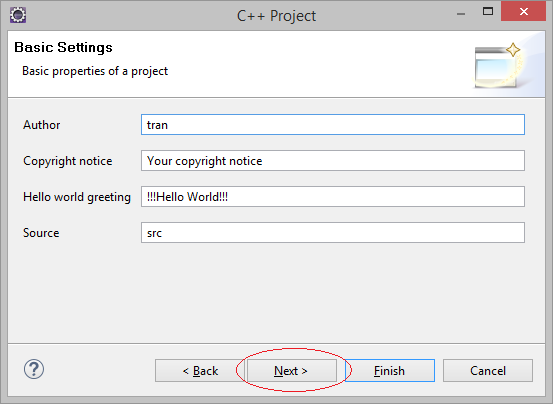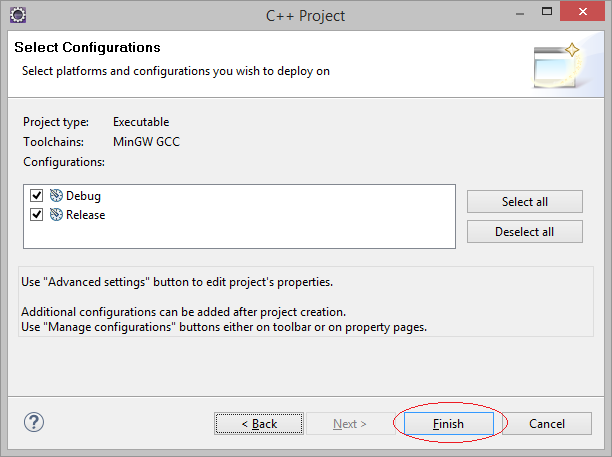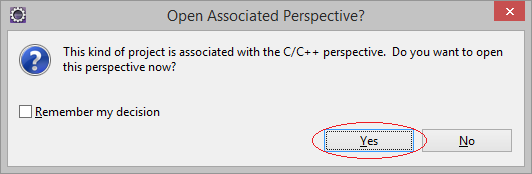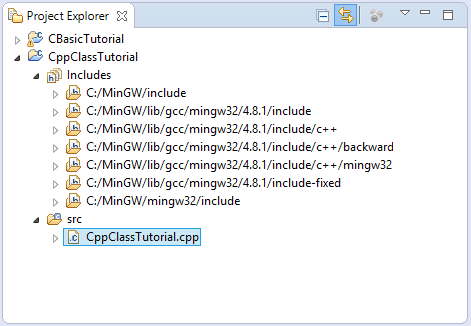Your project has been created with one available source file cpp, we will explain the structure of the source file later.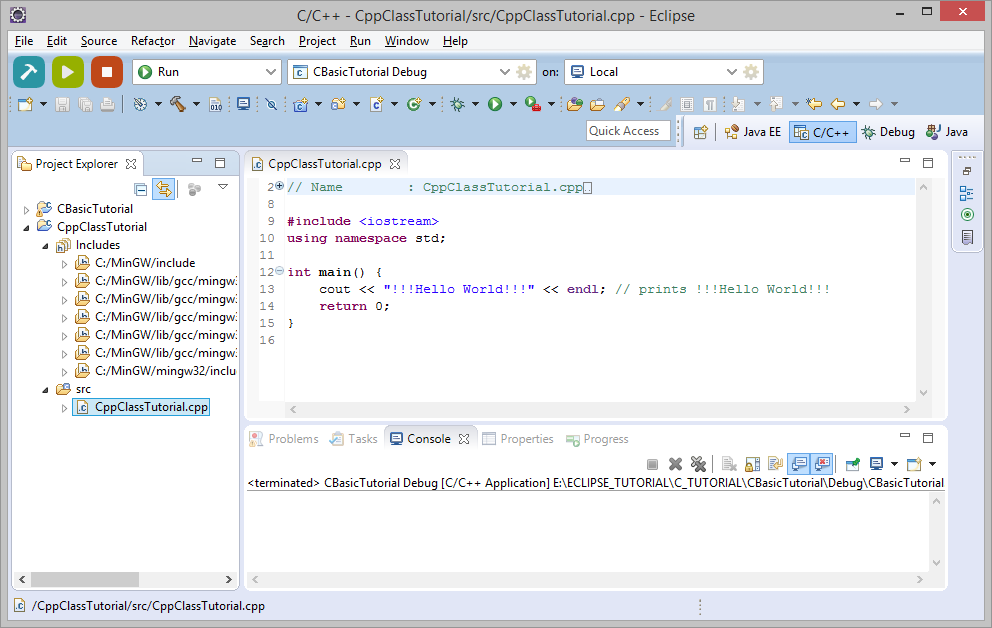## 4- Run C++ applications

In the first, you need to compile your project. Click on the Project and  select "Project/Build All".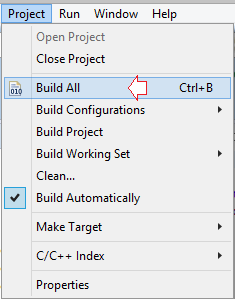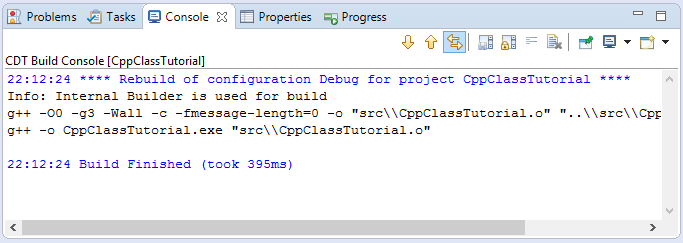Your Eclipse  may be include some project, you need to add a new configuration to run the current Project.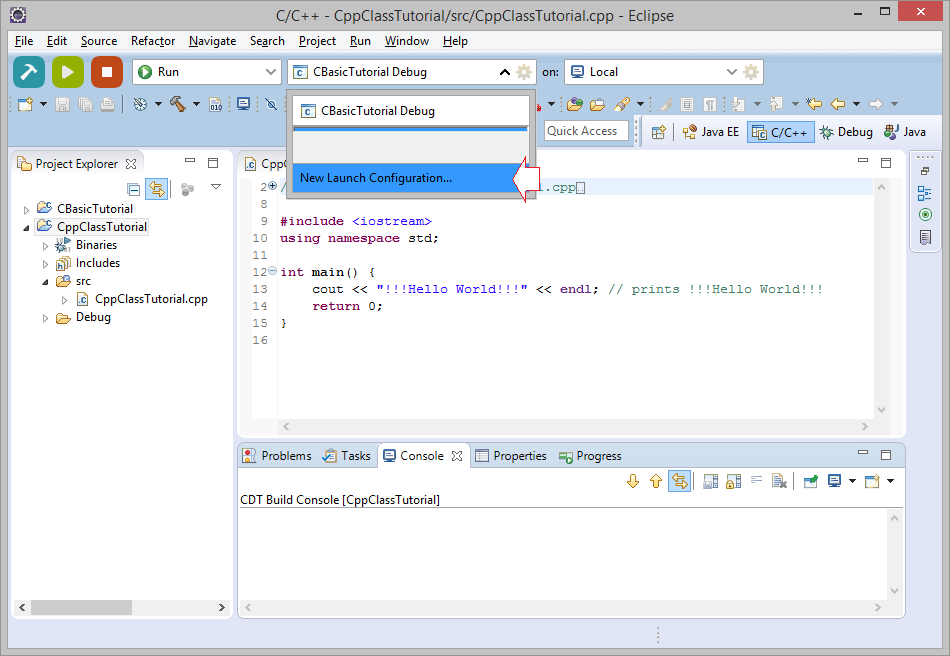You're programming, you should select the running mode is DEBUG.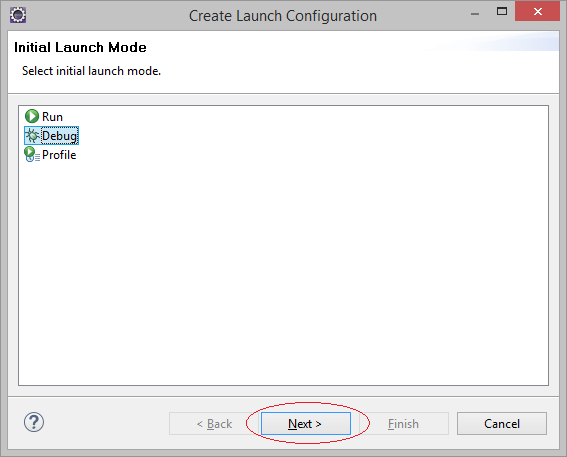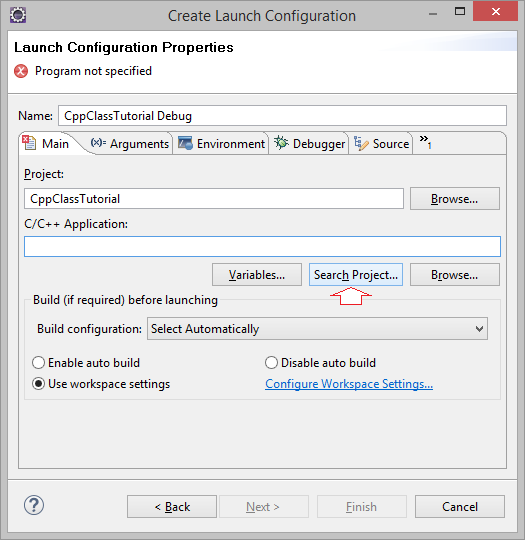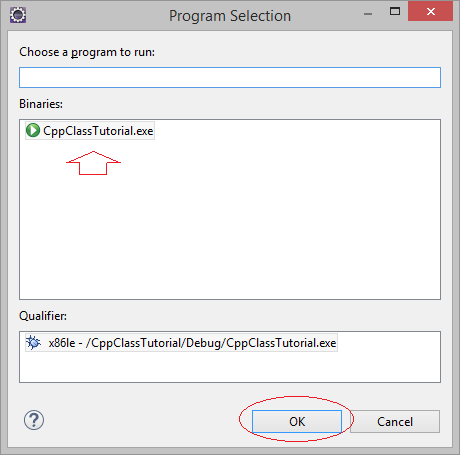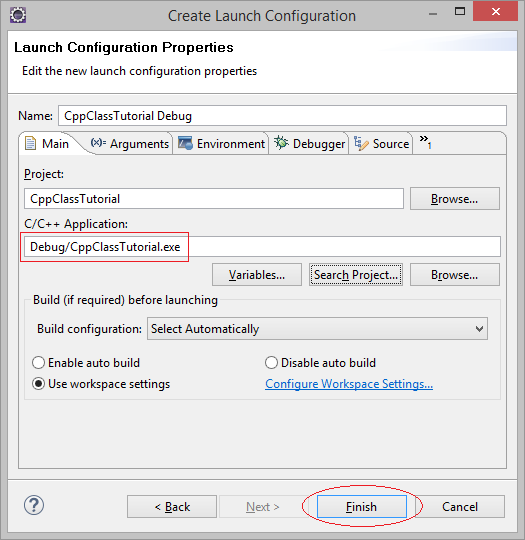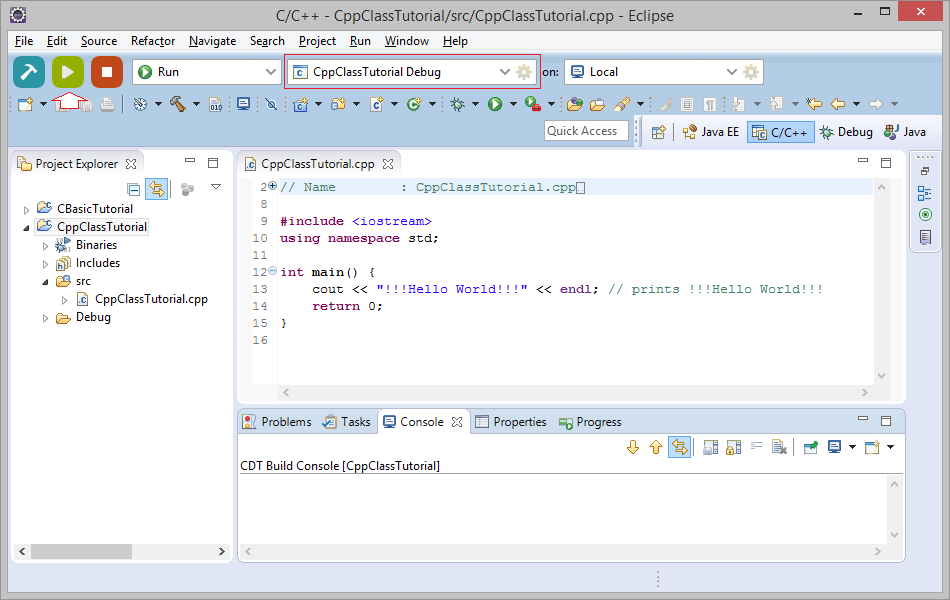## 5- Explain structure of C++ program

Above you have run a simple C++ program, it displays a string to the screen. In C, to print out a string using printf, but with C++ you use cout, of course all of the C functions continues to be used in C++.
Let's see how to use cout:
```// Cout command used to print a string to the screen:

cout << "One";

// Print a string "One " and next is string "Two ".

cout << "One "  << "Two ";

// Print a string "One " next string "Two " and next number 3.

cout << "One " << "Two "  <<  3 ;

// Print a string "One" and next is newline character.

cout << "One" << endl;
```
• TODO

The purpose of C++ is  to object-oriented programming in C programming language. And class  is a concept of C++. Class is a blueprint of (to create) objects.

For simple and easy to understand, I will use the class to model a rectangle with a length and height, and a function to calculate the area of the rectangle.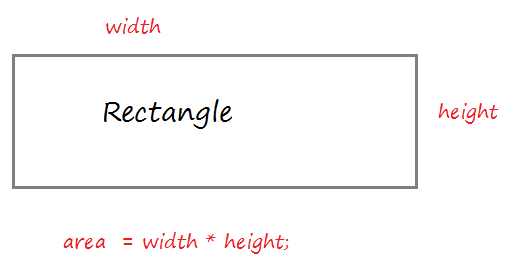Create a resource file Rectangle.cpp: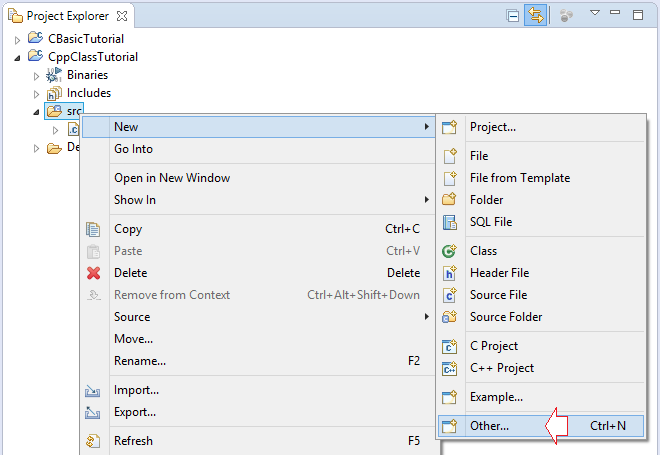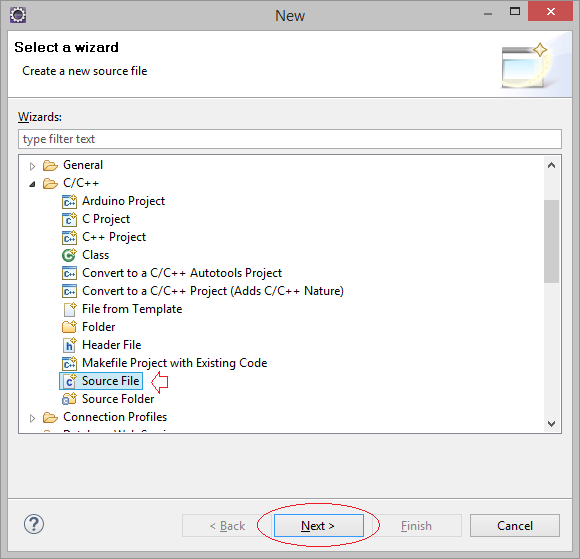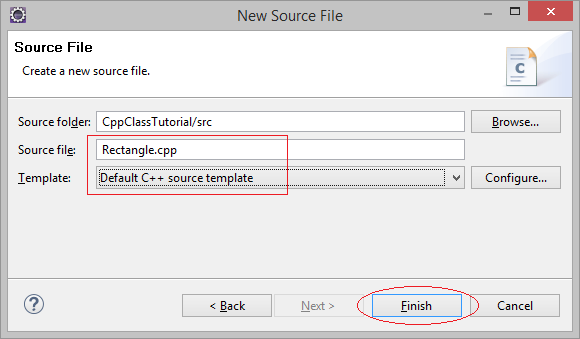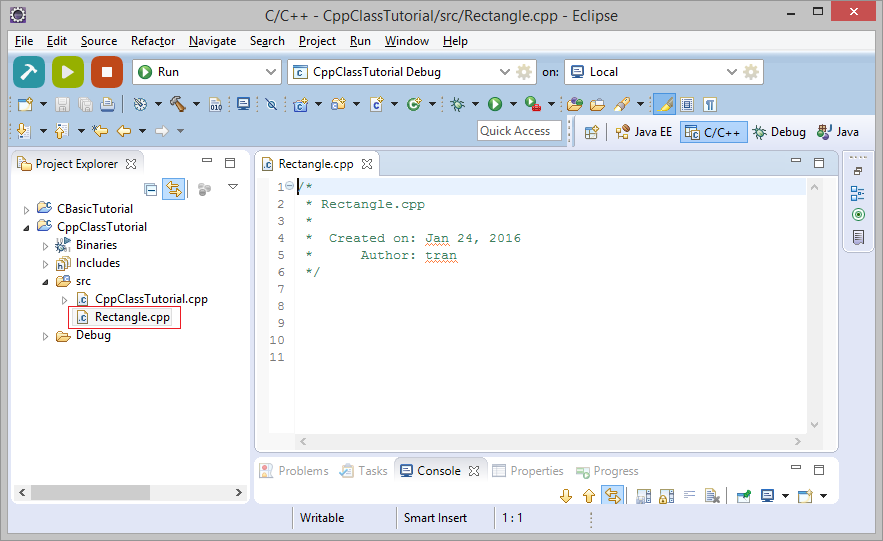Code:
Rectangle.cpp
```#include <iostream>
using namespace std;

namespace mynamespace {

class Rectangle {

private:
int width;
int height;

public:
Rectangle();
Rectangle(int, int);

public:
int getArea() {
return  width * height ;
}
int getWidth() {
return width;
}
int getHeight()  {
return height;
}
};

Rectangle::Rectangle() {
width = 10;
height = 5;
}

Rectangle::Rectangle(int a, int b) {
width = a;
height = b;
}
}

// Declare use libraries located in the namespace 'mynamespace'.
// (Including Rectangle (Because it is in this namespace) ).
using namespace mynamespace;

int main() {

// Create a Rectangle 'rect1' from Constructor Rectangle(int, int).
// Value 5 is assigned to the width, the value 4 is assigned to the height.
Rectangle rect1(5, 4);

// Create a Rectangle 'rect2' from default Contructor Rectangle().
// width, height are assigned default values
Rectangle rect2;

cout << "rect1:" << endl;
// Call method to get with
cout << " width: " << rect1.getWidth() << endl;
// Call method to get heght
cout << " height: " << rect1.getHeight() << endl;

// Call the method to calculate the area.
cout << " area: " << rect1.getArea() << endl;

cout << "rect2:" << endl;
cout << " width: " << rect2.getWidth() << endl;
cout << " height: " << rect2.getHeight() << endl;
cout << " area: " << rect2.getArea() << endl;
return 0;
}
```
Note: The C/C++ allows only one main() function in the entire project, so you need to rename the other main() function into main_xxx() before running this example.
Running the example: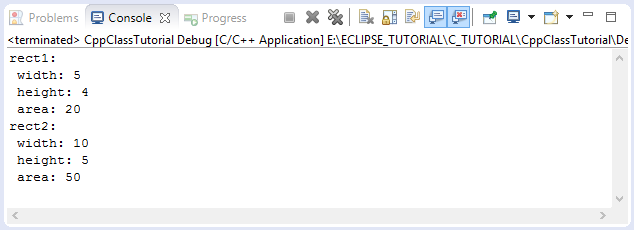Note: There are some keywords like private, protected, public can be located before field, method or a constructor. Temporarily we do not talk about them, it is the access modifier which will be covered in my next tutorial:
Now you should see the explanation of the Class, it's very important.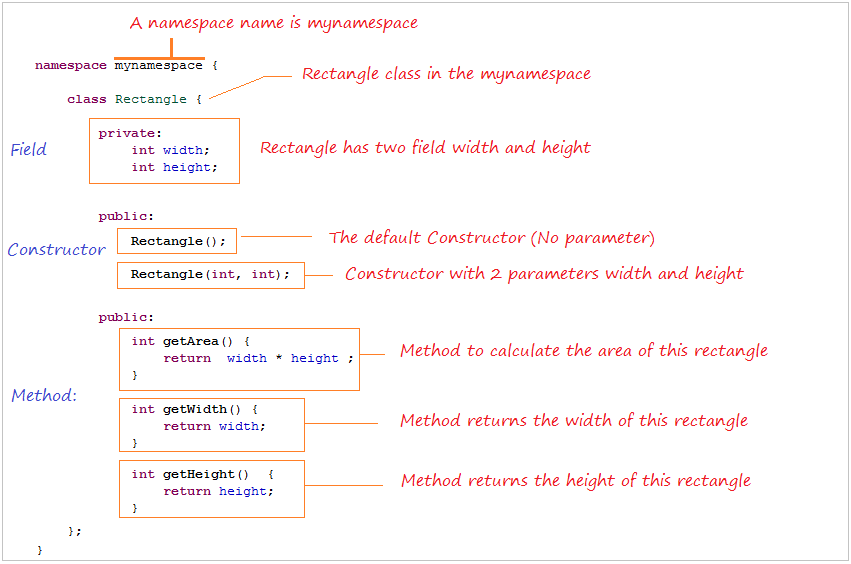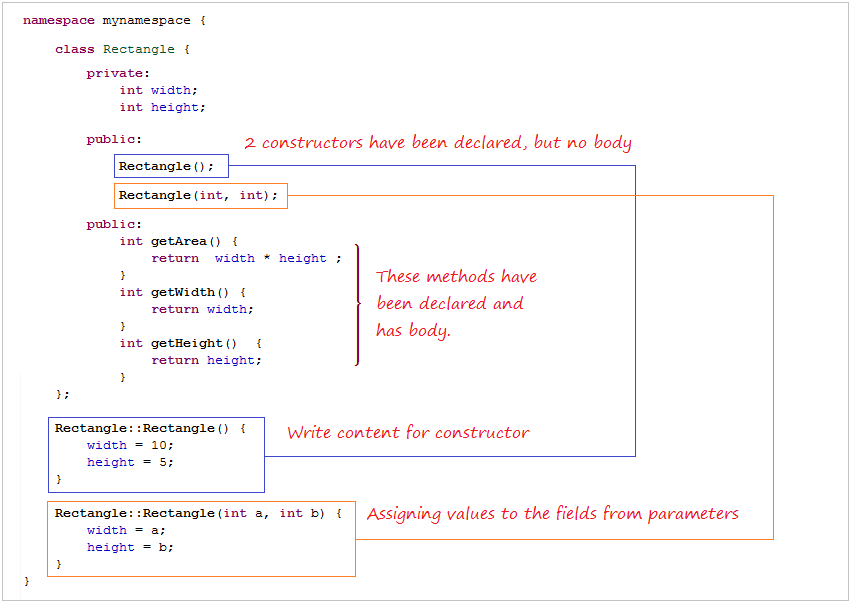## 7- C++ Class example (2)

Next we see an example in C++ Class, which declared all the constructor and methods are in class.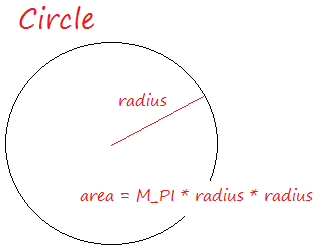Circle.cpp
```#include <iostream>
#include <math.h>
using namespace std;

namespace mynamespace {

class Circle {

private:

public:
Circle() {
}
// Constructor with one parameter.
// Assign value for radius field.
Circle(int r) {
}

public:
float getArea() {
// M_PI is a constant defined in <math.h>
}
// (The content of this method is written in another place)

// Method to assign a new value for the radius
}
};

}
}

// Declare to use the namespace 'mynamespace'.
using namespace mynamespace;

int main() {

// Create a Circle with radius = 5.
Circle circle1(5);

// Create a Circle with defalt radius (100).
Circle circle2;

cout << "Circle 1:" << endl;
cout << " area: " << circle1.getArea() << endl;

cout << "Circle 2:" << endl;
cout << " area: " << circle2.getArea() << endl;

// Set new value for the radius.

cout << "Circle 2 (After set new Radius):" << endl;
cout << " area: " << circle2.getArea() << endl;

return 0;

}
```
Running the example: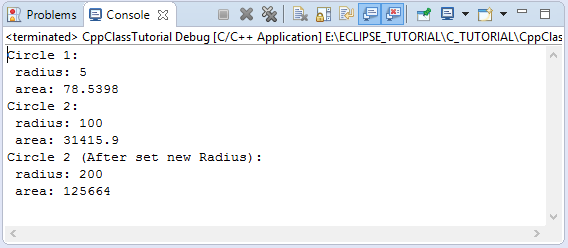## 8- C++ Class example (3)

When you create a class with Wizard of  Eclipse, it will create 2 files named ClassName.h and ClassName.cpp . In which ClassName.h declare that your class includes fields, methods and the Constructor. And their contents will be written in ClassName.cpp.
Let's look at an example, you have created Person class on Eclipse with the help of the Wizard.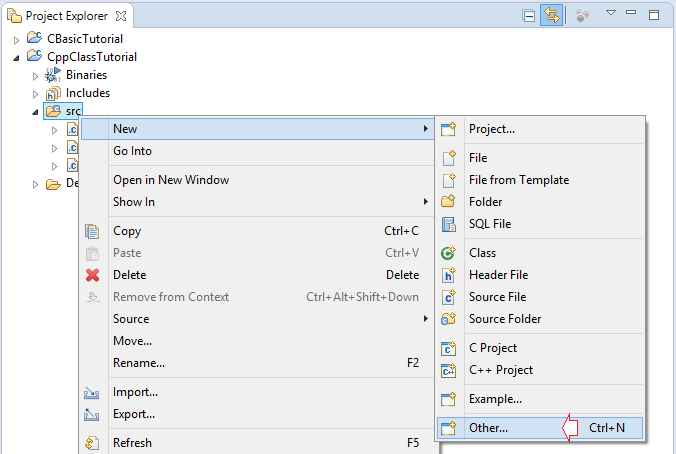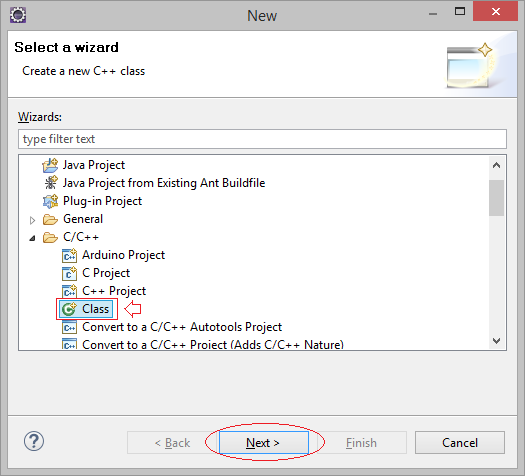Create a class named Person and located in mynamespace namespace.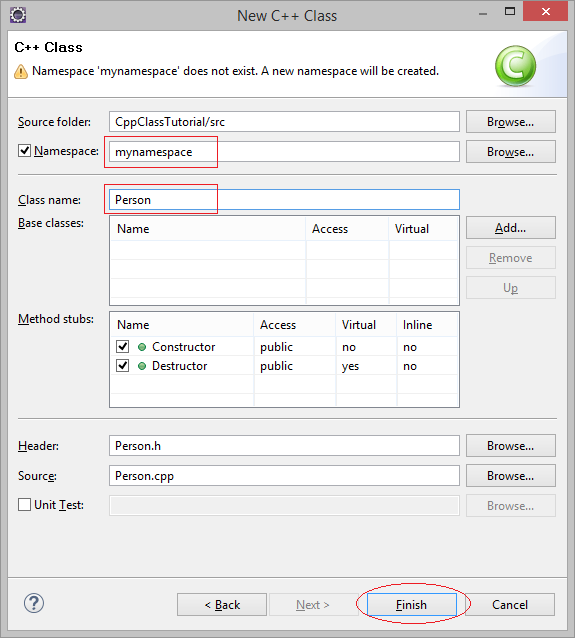Person class has been created, it includes  Person.cpp &  Person.h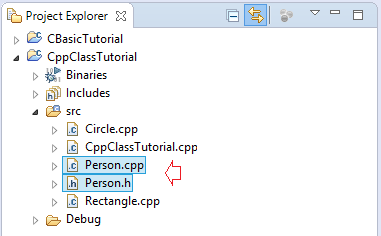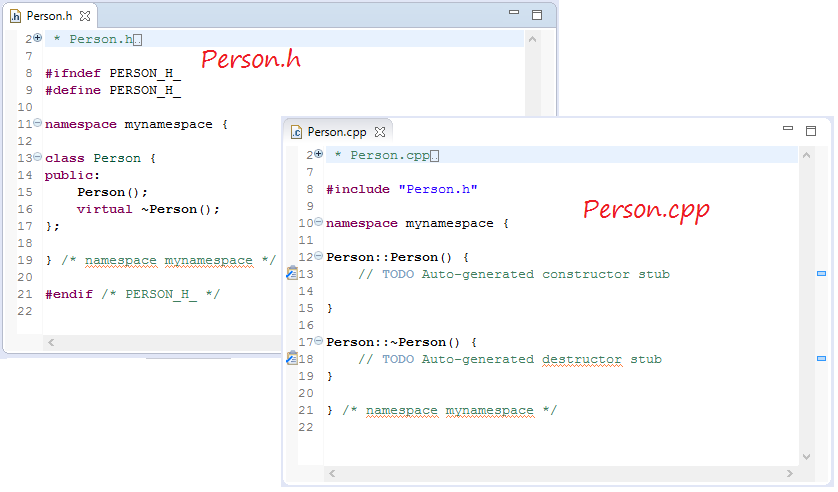Now edit the code of Person.h & Person.cpp:
Person.h
```#ifndef PERSON_H_
#define PERSON_H_

// Using string
#include <string>

// Using namespace std (to use string, cout,..)
using namespace std;

namespace mynamespace {

class Person {

private:
// A field
string name;

public:
// Default constructor
Person();
// Constructor with one parameter.
Person(string);

// Destructor.
virtual ~Person();

public:
// Method return name of person.
string getName();
// Set new name for person.
void setName(string);

};

}

#endif
```
Person.cpp
```// Inclure Person.h
#include "Person.h"
#include <iostream>
#include <string>

using namespace std;

namespace mynamespace {

Person::Person() {
// Default name.
name = "Anonymous";
}

Person::Person(string name) {
// Assign value to field of Person.
// Using this -> name to refers to name field of Person.
// (To avoid confusion with the parameter 'name').
this-> name = name;
}

// Destructor, same name with name of class and has no parameters.
Person::~Person() {
cout<< "Destructor called" <<endl ;
}

// Content of method getName().
string Person::getName()  {
return this-> name;
}

// Set new name for person.
void Person::setName(string newName)  {
// Assign new name.
name = newName;
}

}
```

### Destructor

The object created, and no longer used, it would be removed from the computer's memory, just before it is removed, the Destructor will be called. Normally, when you create an object and use other resources in the system, such as opening file to read,  you can release the file in object's destructor.
You can use  Person class on other source file.
PersonTest.cpp
```#include "Person.h"
#include <iostream>
#include <string>

using namespace std;

using namespace mynamespace ;

int main() {

// Create a Person object.
Person billGate("Bill Gate");

cout << "billGate.getName(): " << billGate.getName() << endl;

// Create a Person from default contructor.

Person aPerson ;

cout << "aPerson.getName(): "<< aPerson.getName() << endl;

cout << "aPerson set new name" << endl;

// Set new name for aPerson.
aPerson.setName("Markus Hess");

cout << "aPerson.getName(): " << aPerson.getName() << endl;

return 0;

}
```
Running the example: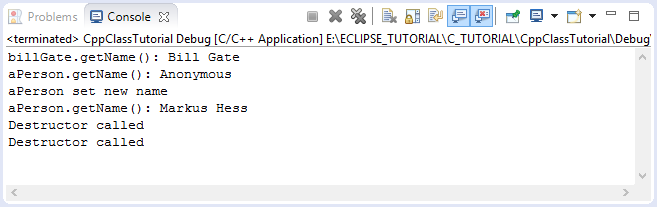#### View more Tutorials:

Maybe you are interested

These are online courses outside the o7planning website that we introduced, which may include free or discounted courses.

•C++ For Absolute Beginners : The Starter Guide
•Learn C++ Programming Mini Course - Power of Animation
•Learning C++ Memory Management
•How to Program in C++ from Beginner to Professional
•Flappy Bird Clone - The Complete SFML C++ Game Course
•Learn C++ by Creating
•Learn C++ Programming The Easy Way
•A Complete Introduction to the C++ Programming Language
•Learn C++ Game Development
•C, C++, Java; A Programming MegaPrimer for Beginners
•Concepts of Object Oriented Programming with C++
•Learn and Understand C++
•Modern OpenGL C++ 3D Game Tutorial Series & 3D Rendering
•Windows 10 C++ App Development for Startups - C++ Simplified
•C++ Intermediate: Build C++ Programs